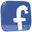Compare genotypes and creatures

Is there any built in functionality to compare two genotypes? I notice when the mutation is performed it includes something like "3.1% mutation of...".
How is that calculated? Is the code available?

Forums:### Comparing individuals can be

Comparing individuals can be performed on genetic and phenetic levels.

The "3.1% mutation of..." is the genetic level. Each genetic encoding has their dedicated genetic operators (mutation, crossover, and others). These operators can provide information about how many percent of genome they have modified (see the GenoOperators class from SVN). So it is up to these operators to provide (or not) this information. As far as I remember, for f1 mutation this is computed as (the number of characters added and deleted)/(number of characters in original genotype).

The phenetic similarity is a separate algorithm that compares pairs of individuals (3D constructs and NNs) and estimates how much they differ.

### Ah yes I see, so that would

Ah yes I see, so that would only work during the mutation - not for comparing two separate Genotypes.

Can you tell me anything about how the evaluateDistance() function calculates a value?### The following papers describe

The following papers describe how the structural dissimilarity is computed:
http://www.framsticks.com/bib/Komosinski-and-Kubiak-2001
http://www.framsticks.com/bib/Komosinski-et-al-2001

We have recently extended this approach to consider geometric dissimilarity as well:
http://www.framsticks.com/bib/Komosinski-and-Kubiak-2011

### Just threw this together,

Just threw this together, thought I'd post it in case anyone wants to use it...

```function levDist(str1,str2)
{
var i,j;
var xxx,temp,min;
var str1len = String.len(str1);
var str2len = String.len(str2);
var d=Vector.new();

for (i=0;i<str1len+1;i++)
{
xxx=Vector.new();
}
xxx = d.get(0);

for (j=0;j<str2len+1;j++)

for (j=1;j<str2len+1;j++)
{
for (i=1;i<str1len+1;i++)
{
if (String.substr(str1,i-1,1) == String.substr(str2,j-1,1))
{
xxx = d.get(i-1);
temp = xxx.get(j-1);
xxx=d.get(i);
xxx.set(j,temp);
}
else
{
xxx = d.get(i-1);
temp = xxx.get(j);
temp++;
min=temp;
xxx = d.get(i);
temp = xxx.get(j-1);
temp++;
if (temp<min)min=temp;
/*xxx = d.get(i-1); //include this if you want to allow for substitutions
temp = xxx.get(j-1);
temp++;
if (temp<min)min=temp;*/
xxx=d.get(i);
xxx.set(j,min);
}
}
}
xxx=d.get(str1len);
return(xxx.get(str2len));
}
```

Certainly not the nicest code ever, but it required a 2D array which explains all the vectors.
So if anyone wants to check the Levenshtein distance between two genotypes just use this!
http://en.wikipedia.org/wiki/Levenshtein_distance### This can be quite useful! For

This can be quite useful! For f1, it would make sense to first remove whitespaces from compared genotypes.

See also `String.diff()`.